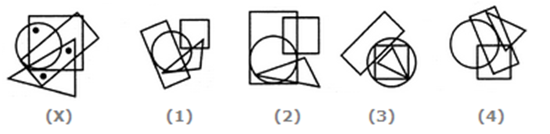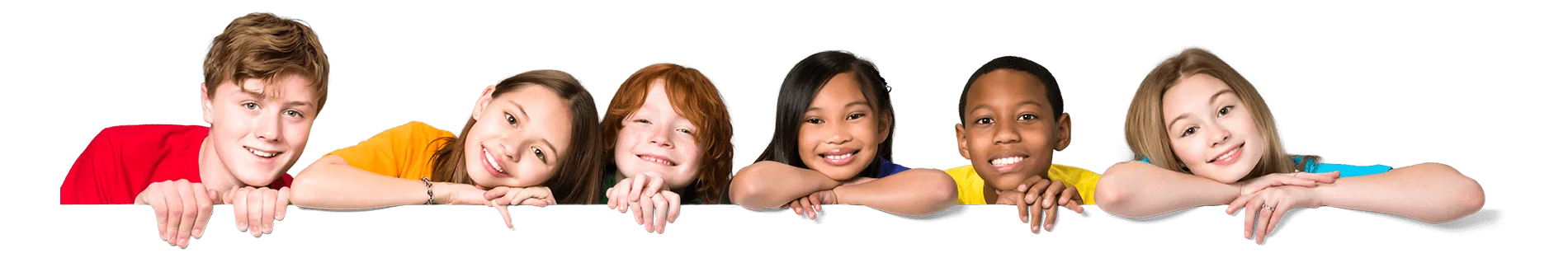Latest Results:

# Free 11 Plus Non Verbal Reasoning sample papers

Solve this Question

#### In which answer figure the question figure is embedded?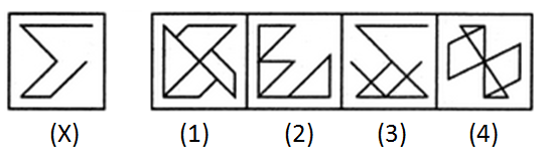1. 1 2. 2 3. 3 4. 4

Solve this Question

#### Find the number of triangles in the given figure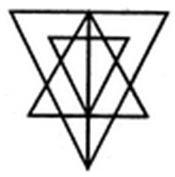1. 27 2. 25 3. 23 4. 21

Solve this Question

#### Determine the figure similar to question figures.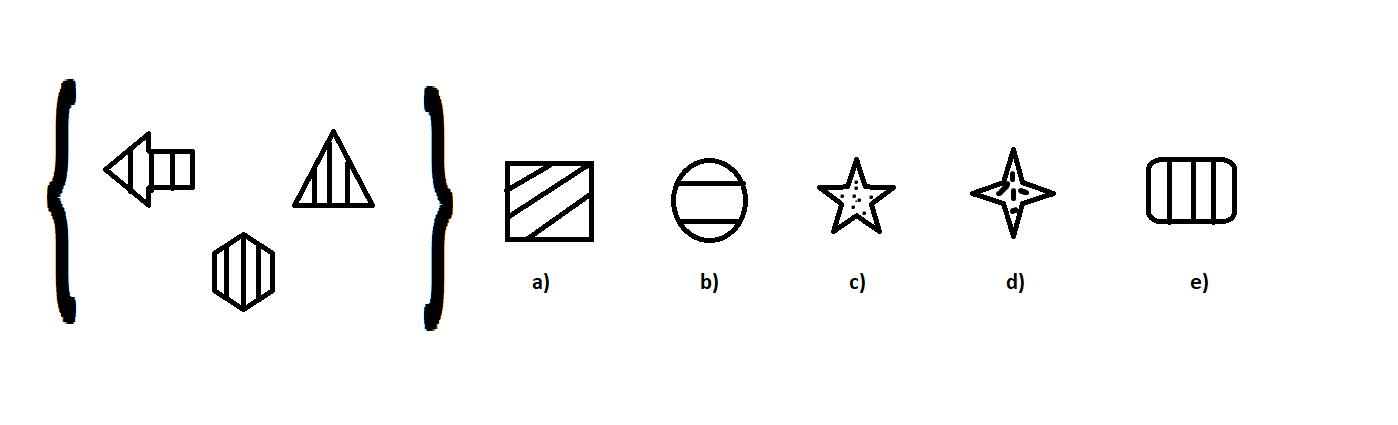1. a 2. b 3. c 4. d 5. e

Solve this Question

#### What is the mirror image of the question figure, reflecting it along a plane placed on the right side of the question figure?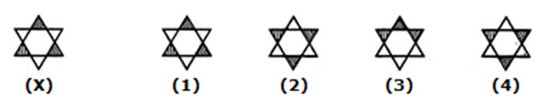1. 1 2. 2 3. 3 4. 4

Solve this Question

#### From the given orientations of a dice, find out the number which is on opposite face of number 2?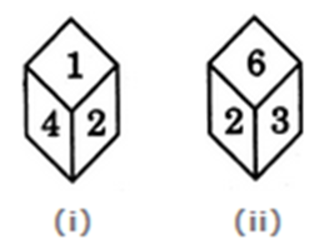1. 1 2. 5 3. 3 4. 6

Solve this Question

#### On observing the first set, what would be the answer figure in the second set?1. a 2. b 3. c 4. d 5. e

Solve this Question

#### By observing the pattern followed in the question figure, complete the pattern.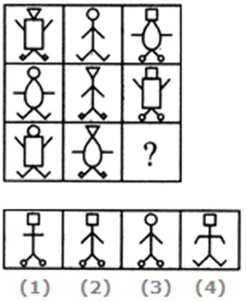1. 1 2. 2 3. 3 4. 4

Solve this Question

#### Combine two or more pieces given below to form a rectangle.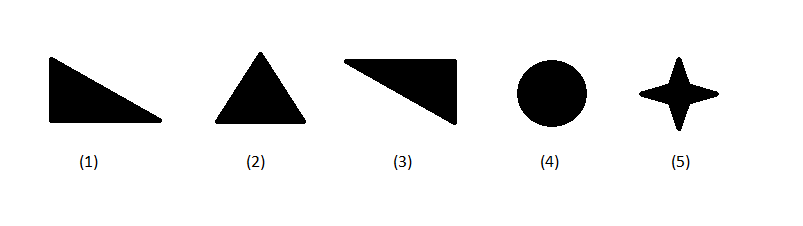1. 1,3 2. 1,3,5 3. 2,3,4 4. 2, 4, 5

Solve this Question

#### Find the number of triangles in the given figure.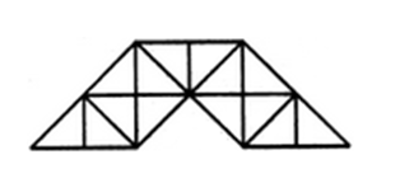1. 23 2. 27 3. 29 4. 31

Solve this Question

#### By observing the pattern followed in the question figure, complete the pattern.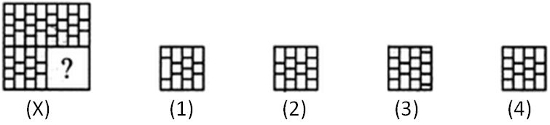1. 1 2. 2 3. 3 4. 4

Solve this Question

#### Group the figures down below into three sets each consisting of 3 figures, which follow the same pattern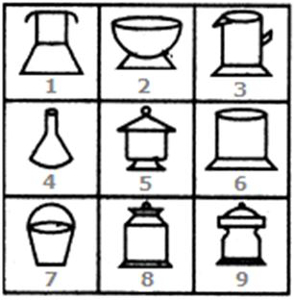1. 1,4,7 ; 2,5,9 ; 3,8,6 2. 2,6,9 ; 1,4,7 ; 5,8,3 3. 1,4,7 ; 2,3,6 ; 5,8,9 4. 3,5,1 ; 4,7,8 ; 6,2,9

Solve this Question

#### Combine two or more pieces given below, to form a closed figure.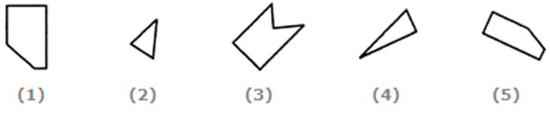1. 1,2,4 2. 1,2,5 3. 2,3,4 4.  2,4,5

Solve this Question

#### By observing the pattern followed in the question figure, what should be the next figure?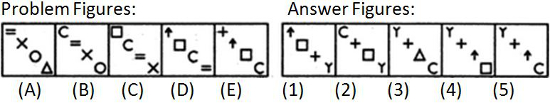1. 1 2. 2 3. 3 4. 4 5. 5

Solve this Question

#### Observe the pattern followed by the figures and find the odd one out from them.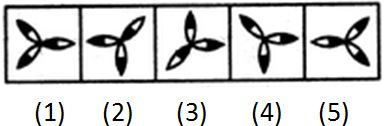1. 1 2. 2 3. 3 4. 4 5. 5

Solve this Question

#### By observing the pattern followed in the question figure, what should be the next figure?1. 1 2. 2 3. 3 4. 4 5. 5

Solve this Question

#### By observing the pattern followed in the question figure, complete the pattern.1. a 2. b 3. c 4. d 5. e

Solve this Question

#### Observe the pattern followed by the figures and find the odd one out from them.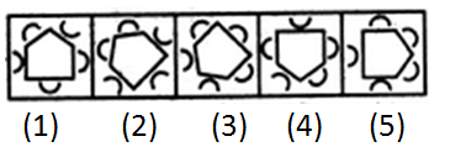1. 1 2. 2 3. 3 4. 4 5. 5

Solve this Question

#### Find the number of triangles in the given figure.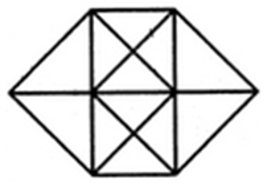1. 20 2. 24 3. 28 4. 32

Solve this Question

#### Determine the figure similar to question figures.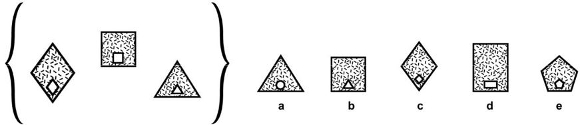1. a 2. b 3. c 4. d 5. e

Solve this Question

#### From amongst the figures marked (1), (2), (3) and (4), select the figure which satisfies the same conditions of placement of the dots as in figure (X)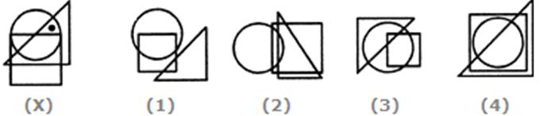1. 1 2. 2 3. 3 4. 4

Solve this Question

#### What is the mirror image of the question figure, on reflecting it along a horizontal plane placed on the right side of the question figure?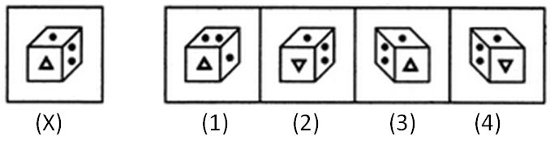1. 1 2. 2 3. 3 4. 4

Solve this Question

#### By observing the pattern of alphabetical codes mentioned below every question figure, what will be the missing code?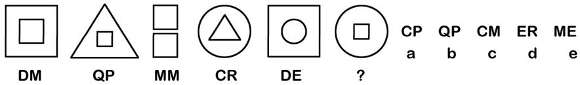1. a 2. b 3. c 4. d 5. e

Solve this Question

#### Observe the relationship between the first two figures, and find the missing figure by applying the same relationship as that of the first two figures.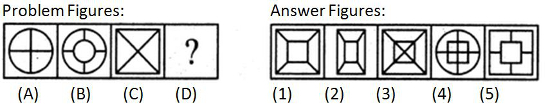1. 1 2. 2 3. 3 4. 4 5. 5

Solve this Question

#### What is the next figure in the series given below?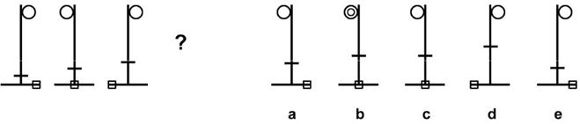1. a 2. b 3. c 4. d 5. e

Solve this Question

#### Observe the relationship between the first two figures, and find the missing figure by applying the same relationship as that of the first two figures.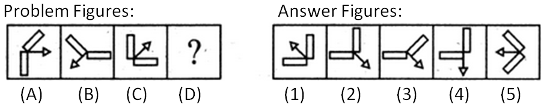1. 1 2. 2 3. 3 4. 4 5. 5

Solve this Question

#### Determine the figure similar to question figures.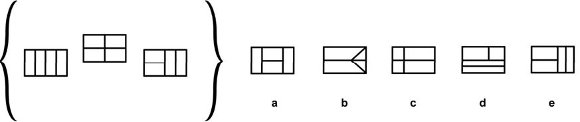1. a 2. b 3. c 4. d 5. e

Solve this Question

#### On observing the first set, what would be the answer figure in the second set?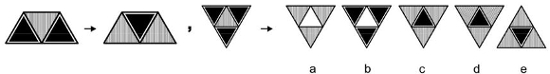1. a 2. b 3. c or d 4. e 5.

Solve this Question

#### Observe the pattern followed by the figures and find the odd one out from them.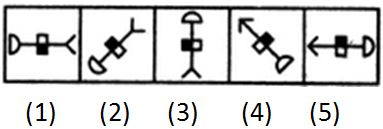1. 1 2. 2 3. 3 4. 4 5. 5

Solve this Question

#### Observe the pattern followed by the figures and find the odd one out from them.1. 1 2. 2 3. 3 4. 4 5. 5

Solve this Question

#### Which of the following letter cannot be on the opposite face of the letter 'e'?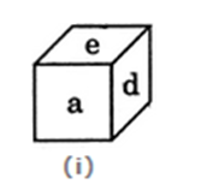1. a 2. b 3. c 4. f

Solve this Question

#### If you unfold the question figure present at the left, as directed in the question figures present to the left of it, what is the answer figure you arrive at?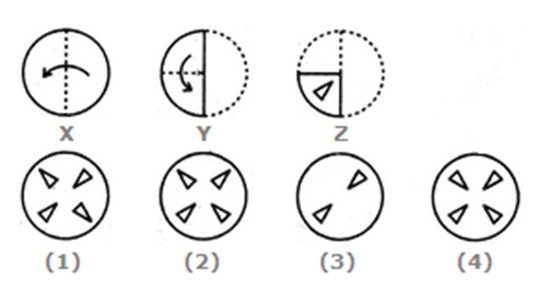1. 1 2. 2 3. 3 4. 4

Solve this Question

#### If you unfold the question figure present at the left, as directed in the question figures present to the left of it, what is the answer figure you arrive at?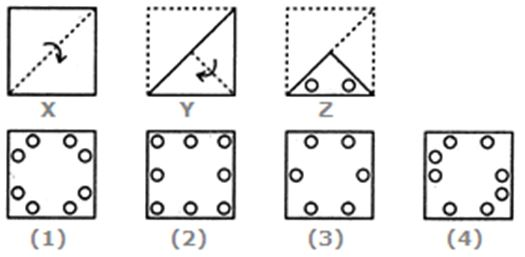1. 1 2. 2 3. 3 4. 4

Solve this Question

#### What is the mirror image of the question figure, on reflecting it along a horizontal plane placed on the right side of the question figure?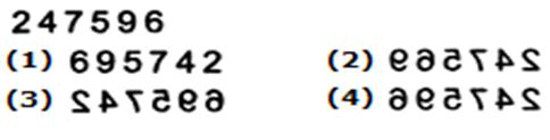1. 1 2. 2 3. 3 4. 4

Solve this Question

#### Group down the figures into 3 sets each consisting of 3 figures, which are of same kind.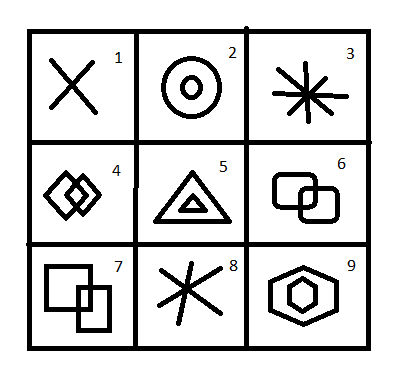1. 1,3,9; 2,5,8; 4,6,7 2. 1,3,8; 2,5,9; 4,6,7 3. 1,3,8; 2,4,9; 5,6,7 4. 1,7,8; 2,5,9; 3,4,6

Solve this Question

#### What is the figure you arrive at, by folding the paper to form a cube?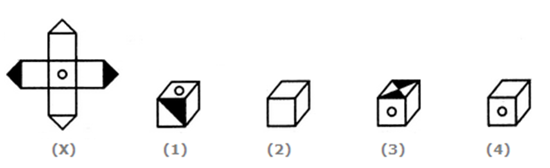1. 1 2. 2 3. 3 4. 4

Solve this Question

#### By observing the pattern followed in the question figure, complete the pattern.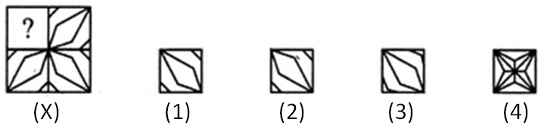1. 1 2. 2 3. 3 4. 4

Solve this Question

#### By observing the pattern of alphabetical codes mentioned below every question figure, what will be the missing code?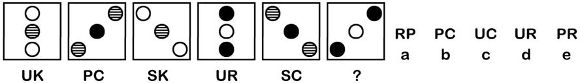1. a 2. b 3. c 4. d 5. e

Solve this Question

#### Group the figures down below into three sets each consisting of 3 figures, which follow the same pattern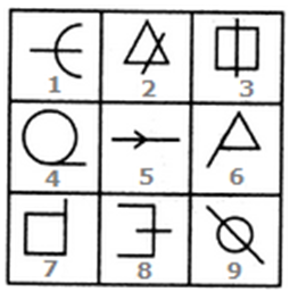1. 1,3,9 ; 2,5,8 ; 4,6,7 2. 1,5,8 ; 4,6,7 ; 2,3,9 3. 2,5,9 ; 1,3,8 ; 2,6,7 4. 1,8,9 ; 4,6,7 ; 2,3,5

Solve this Question

####1. 1,5,7 ; 2,4,6 ; 3,9,8 2. 1,5,7 ; 2,4,8 ; 3,6,9 3. 1,4,7 ; 2,5,8 ; 3,6,9 4. 1,7,9 ; 3,5,8 ; 2,4,6

Solve this Question

#### By observing the pattern followed in the question figure, what should be the missing part?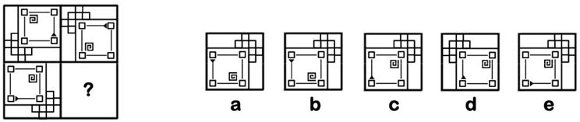1. a 2. b 3. c 4. d 5. e

Solve this Question

#### Rotate the question figure in the desired direction and determine the answer figure.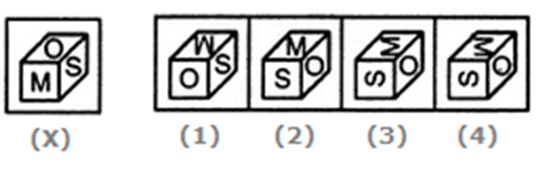1. 1 2. 2 3. 3 4. 4

Solve this Question

#### By observing the pattern followed in the question figure, what should be the missing part?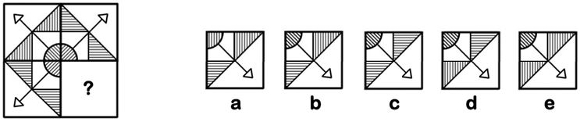1. a 2. b 3. c 4. d 5. e

Solve this Question

#### On observing pattern in the first set, what would be the answer figure in the second set?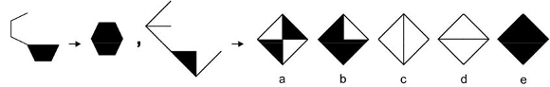1. a 2. b 3. c 4. d 5. e

Solve this Question

#### By observing the pattern of alphabetical codes mentioned below every question figure, what will be the missing code?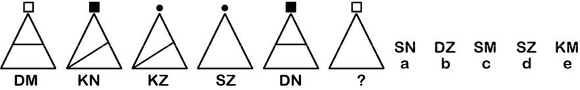1. a 2. b 3. c 4. d 5. e

Solve this Question

#### By observing the pattern followed by the question figures, what will be the missing figure?(consider figure A, B as one set and C, D as one set)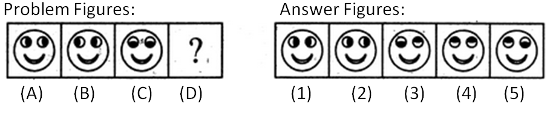1. 1 2. 2 3. 3 4. 4 5. 5

Solve this Question

#### In which answer figure, the question figure is embedded?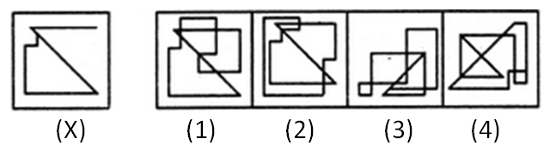1. 1 2. 2 3. 3 4. 4

#### From amongst the figures marked (1), (2), (3) and (4), select the figure which satisfies the same conditions of placement of the dots as in figure (X)